# 05-pandas索引切片讀取數據缺失數據處理

## 引入

numpy已經能夠幫助我們處理數據，能夠結合matplotlib解決我們數據分析的問題，那么pandas學習的目的在什么地方呢？ numpy能夠幫我們處理處理數值型數據，但是這還不夠 很多時候，我們的數據除了數值之外，還有字符串，還有時間序列等 比如：我們通過爬蟲獲取到了存儲在數據庫中的數據 比如：之前youtube的例子中除了數值之外還有國家的信息，視頻的分類(tag)信息，標題信息等 所以，numpy能夠幫助我們處理數值，但是pandas除了處理數值之外(基于numpy)，還能夠幫助我們處理其他類型的數據。

### 什么是pandas？

pandas是一個Python軟件包，提供快速，靈活和富于表現力的數據結構，旨在使使用“關系”或“標記”數據既簡單又直觀。它旨在成為在Python中進行實際，真實世界數據分析的基本高級構建塊。此外，其更廣泛的目標是成為任何語言中可用的最強大，最靈活的開源數據分析/操作工具。它已經朝著這個目標邁進了。

### pandas的常用數據類型

1、Series 一維，帶標簽數組

2、DataFrame 二維，Series容器

### (1)Series創建

pandas.Series(data=None, index=None, dtype=None, name=None, copy=False, fastpath=False)

data :類 數組，可迭代，字典或標量值,包含存儲在系列中的數據。在0.23.0版中進行了更改：如果data是dict，則將為Python 3.6及更高版本維護參數順序。

index ：類 數組或索引（1d）值必須是可散列的，并且與data的長度相同。允許使用非唯一索引值。如果未提供，則默認為RangeIndex（0，1，2，…，n）。如果同時使用了字典和索引序列，則索引將覆蓋在字典中找到的鍵。

dtype ： STR，numpy.dtype，或ExtensionDtype，可選

copy： bool，默認為False,copy輸入數據。

import pandas as pd
import numpy as np
t = pd.Series(np.arange(12),index= list("asdfghjklpoi"))
print(t)print(type(t))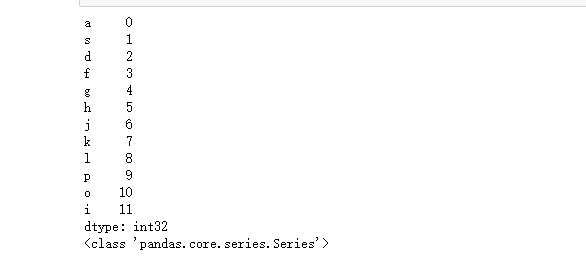注意幾個問題：pd.Series能干什么，能夠傳入什么數據類型讓其變為series結構。index是什么，在什么位置，對于我們常見的數據庫數據或者ndarray來說，index到底是什么如何給一組數據指定index。

c = {"name":"lishuntao","age":18,"gender":"boy"}
t1 = pd.Series(c)
print(t1)
print(type(t1))
print(t1["name"])
print(t1["gender"])

import numpy as np
import pandas as pd

a = {"a":12,"name":"lishuntao","c":"xiaoc","age":18,"gender":"man"}

t1 = pd.Series(a)
print(t1)
print(type(t1))
t2 = pd.Series(a,index=list("abcdf"))
print(t2)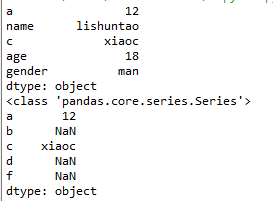numpy中的nan為float，pandas會自動根據數據類型更改series的dtype類型。

## Series切片和索引

import numpy as np
import pandas as pd

a = {"a":12,"name":"lishuntao","c":"xiaoc","age":18,"gender":"man"}

t1 = pd.Series(a)
print(t1)
print(t1[:2])
print(t1)
print(t1[["a","c","gender"]])
print(t1[0:5:2])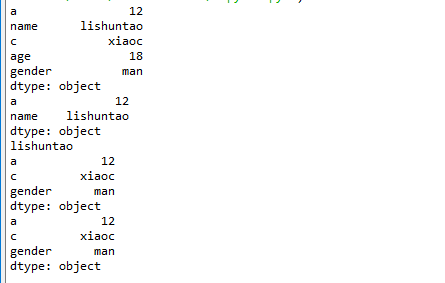import numpy as np
import pandas as pd

a = np.arange(12)
t1 = pd.Series(a)
print(t1)
print(t1[t1>9])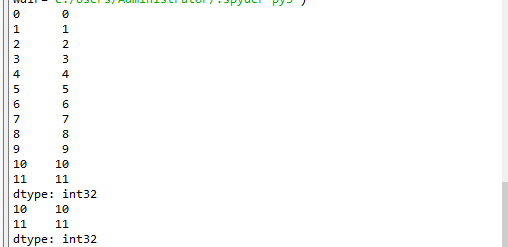### Series的索引和值

import numpy as np
import pandas as pd

a = np.arange(12)
t1 = pd.Series(a)
#print(t1)
print(t1.index)
print(t1.values)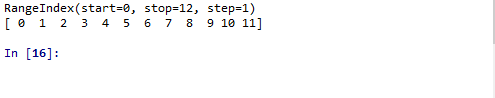import numpy as np
import pandas as pd

a = np.arange(12)
t1 = pd.Series(a)
print(t1)
print(type(t1.index))
print(type(t1.values))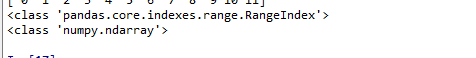Series對象本質上有兩個數組構成，一個數組構成對象的鍵（index，索引），一個數組構成對象的值（values），鍵--->值。

ndarray的很多方法都可以運用與series類型，比如argmax，clip

series具有where方法，但是結果卻不同（下面是官方文檔給出）

 Series.where(self, cond[, other, inplace, …]) Replace values where the condition is False.

a = np.arange(12)
t1 = pd.Series(a)
print(t1)
#替換條件是False的情況 下面兩個結果一樣
print(t1.where((t1>8),1))print(pd.Series.where(t1,(t1>4),1))

## pandas之讀取外部數據

現在假設我們有一個組關于狗的名字的統計數據，那么為了觀察這組數據的情況，我們應該怎么做呢？

數據來源：https://www.kaggle.com/new-york-city/nyc-dog-names/data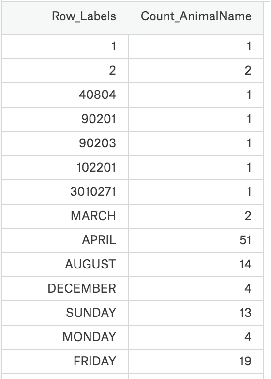import numpy as np
import pandas as pd
print(t2)
print(type(t2))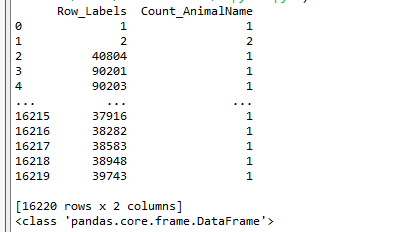## (2)DataFrame的創建

pd.DataFrame（data,index,columns,dtype,copy）

import numpy as np
import pandas as pd
t2 = pd.DataFrame(np.arange(12).reshape(3,4))
print(t2)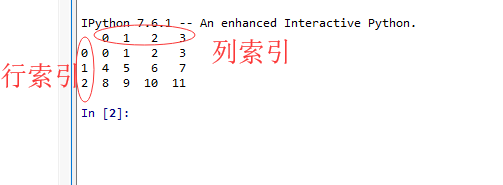從上面我們可以看出DataFrame對象既有行索引，又有列索引

自定義索引標簽：

t2 = pd.DataFrame(np.arange(12).reshape(3,4),index=list("abc"),columns=list("WXYZ"))
print(t2)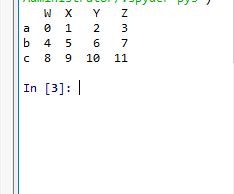### DataFrame的基礎屬性

t2 = pd.DataFrame(np.arange(12).reshape(3,4),index=list("abc"),columns=list("WXYZ"))
print(t2)
print(t2.shape)#顯示行數，列數
print(t2.dtypes)#顯示的是列數據類型
print(t2.ndim)#數據維度2（0,1）
print(t2.index)#行索引
print(t2.columns)#列索引 Index(['W', 'X', 'Y', 'Z'], dtype='object')
print(t2.values)#對象值，二維ndarray的數組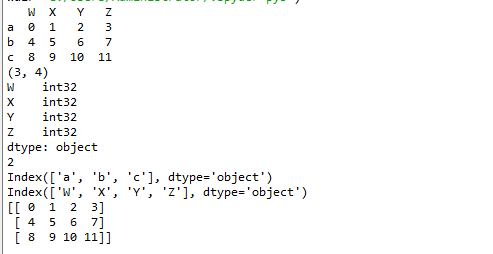### DataFrame整體情況查詢

t2 = pd.DataFrame(np.arange(12).reshape(3,4),index=list("abc"),columns=list("WXYZ"))
print(t2)
print(t2.tail(2))#顯示末尾幾行，默認5行
print(t2.info())#相關信息瀏覽：行數，列數，列索引，列非空值個數，列類型，列類型，內存占用
print(t2.describe())#快速綜合統計結果：計數，均值，標準差，最大值，四分位數，最小值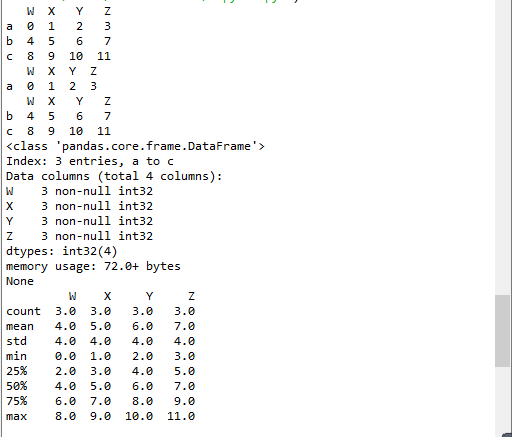動手：那么回到之前我們讀取的狗名字統計的數據上，我們嘗試一下剛剛的方法

pd.DataFrame.sort_values(by="Count_AnimalName",ascending=False)#ascending=True升序排序 by是對那一列排序 輸入列索引鍵
t2 = pd.read_csv("F:\BaiduNetdiskDownload\youtube_video_data\dogNames2.csv")
print(t2)

t3 = t2.sort_values(by="Count_AnimalName",ascending=False)
print(t3)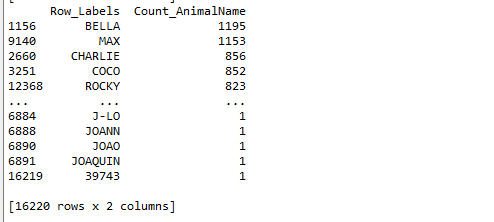### pandas之取行或者列

剛剛我們知道了如何給數據按照某一行或者列排序，那么現在我們想單獨研究使用次數前100的數據，應該如何做？

t2 = pd.read_csv("F:\BaiduNetdiskDownload\youtube_video_data\dogNames2.csv")
print(t2)

t3 = t2.sort_values(by="Count_AnimalName",ascending=False)
print(t3[:100])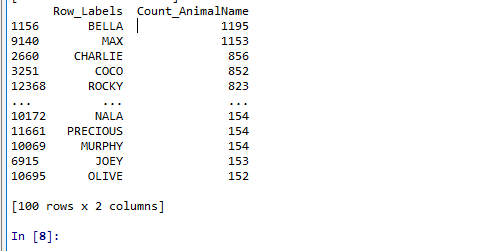我們具體要選擇某一列該怎么選擇呢？t2[" Count_AnimalName "]

### pandas之loc取行數據

1、t2.loc 通過標簽索引行數據（標簽）

print(t2.loc["a","W"])
print(t2.loc["a",["W","Y"]])
print(type(t2.loc["a",["W","Y"]]))
print(t2.loc[["a","b"],["Z","Y"]])
print(t2.loc[:"c",:"Y"])
print(t2.loc["a":"b",["W","Z"]])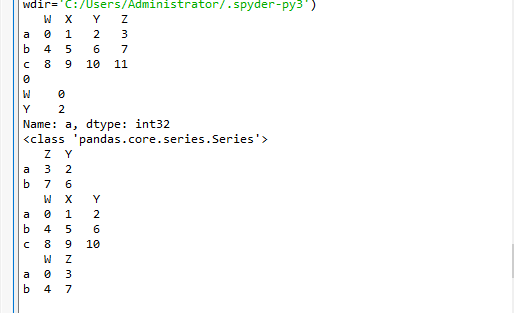2、t2.iloc 通過位置獲取行數據（位置）

import numpy as np
import pandas as pd

t2 = pd.DataFrame(np.arange(12).reshape(3,4),index=list("abc"),columns=list("WXYZ"))
print(t2)
print(t2.iloc[0:2,0:4])
print(t2.iloc[[0,2],[1,3]])
t2.loc["a","Y"] = 100 #復制操作
print(t2)
t2.iloc[1:2,] = 1000 #復制操作
print(t2)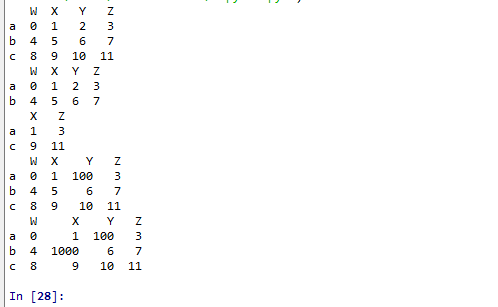### pandas之布爾索引（且，或，&，|，）

回到之前狗的名字的問題上，假如我們想找到所有的使用次數超過800的狗的名字，應該怎么選擇？

print(t2[t2["Count_AnimalName"]>800])

print(t2[(t2["Row_Labels"].str.len()>4)&(t2["Count_AnimalName"]>700)])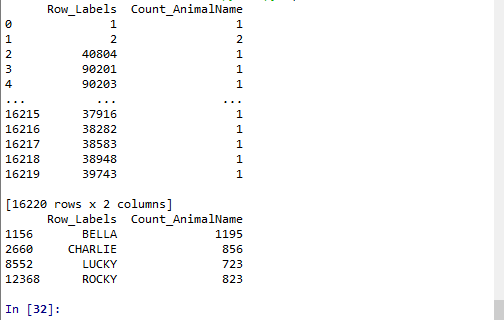#### pandas之字符串方法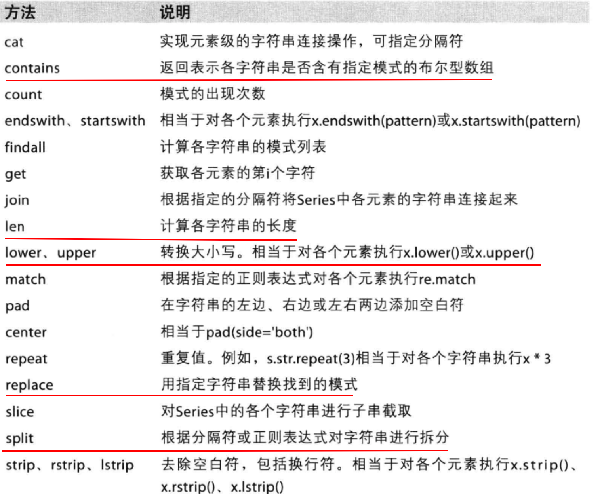### 缺失數據的處理：

觀察這組數據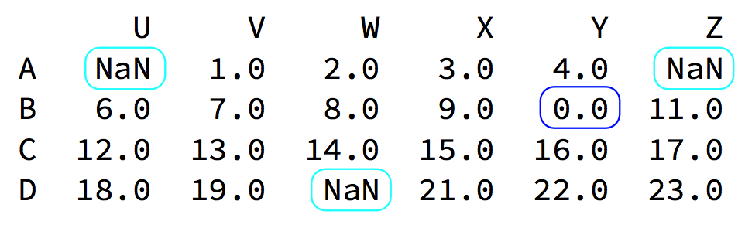我們的數據缺失通常有兩種情況： 一種就是空，None等，在pandas是NaN(和np.nan一樣) 另一種是我們讓其為0（藍色框中）

對于NaN的數據，在numpy中我們是如何處理的？

在pandas中我們處理起來非常容易 判斷數據是否為NaN：pd.isnull(df),pd.notnull(df)

處理方式1：刪除NaN所在的行列

dropna (axis=0, how='any', inplace=False)

處理方式2：填充數據，

t.fillna(t.mean()),t.fiallna(t.median()),t.fillna(0)

處理為0的數據：t[t==0]=np.nan 當然并不是每次為0的數據都需要處理 計算平均值等情況，nan是不參與計算的，但是0會

import numpy as np
import pandas as pd

t2 = pd.DataFrame(np.arange(12).reshape(3,4),index=list("abc"),columns=list("WXYZ"))
#print(t2)
t2.loc[:"b",["W","Y"]] = np.nan
print(t2)
print(pd.isnull(t2))
print(pd.notnull(t2))
#print(t2.dropna(axis=0,how="all",inplace=False))
#any只要含NaN就刪除前面規定的行列，all需要的是行列全部為NAN才能刪除
#填充數據
#print(t2.fillna(t2.mean()))
print(t2)
print(t2.fillna(t2.median()))
print(t2.fillna(0))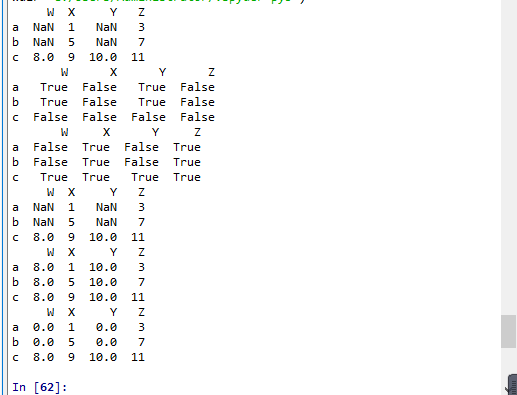posted @ 2019-10-09 10:16 一知.半解 閱讀(...) 評論(...) 編輯 收藏